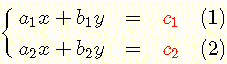# Systems of Equations Solver and Calculator

Two online calculators and solvers for systems of 2 by 2 and 3 by 3 linear equations using Cramer's rule and showing the steps.

## Review Cramer's Rules

The solutions of a systems of a 2 linear equations with two varibles x and y of the formare given by Cramer's rule as follows
$x = \dfrac{D_x}{D} , y = \dfrac{D_y}{D}$
where the
determinant of a 2 by 2 Matrix , $D$, $D_x$ and $D_y$ are defined by

$D = \begin{vmatrix}a_1&b_1\\ a_2&b_2\end{vmatrix} = a_1 b_2 - b_1 a_2$

$D_x = \begin{vmatrix}\color{red}{c_1} & b_1\\ \color{red}{c_2} & b_2\end{vmatrix} = c_1 b_2 - b_1 c_2$

$D_y = \begin{vmatrix}a_1 & \color{red}{c_1}\\ a_2 & \color{red}{c_2}\end{vmatrix} = a_1 c_2 - c_1 a_2$

Camer's rules give a solution for $D \ne 0$.

## Use the Systems of Equations Solver and Calculator to Solve 2 by 2 Syatems of Equations

Enter the coefficients $a_1, b_1, c_1, a_2, b_2 \text{ and } c2$ as defined in the system above and the number of decimal places in the results as decimals and press "Solve System" to obtain the answer, the values of the different determinant and all steps included in the calculations.
This tool can be used to check the solutions of a 2 by 2 system of equations solved by hand. It can also be used, efficiently, to explore 2 by 2 system of equations using different values for the coefficients.

 $a_1$ = 1 $b_1$ = 2 $c_1$ = 3 $a_2$ = 1 $b_2$ = 6 $c_2$ = 5 Decimal Places = 3 $x$ = $y$ =
Steps

,         ,

,

## Systems of Equations Solver and Calculator to Solve 3 by 3 Systems of Equations

Below is a 3 by 3 system of linear equations solver where the system is of the form
$\left\{ \begin{array}{lcl} a_1 x + b_1 y + c_1 z = & \color{red}{d_1}\\ a_2 x + b_2 y + c_2 = & \color{red}{d_2} \\ a_3 x + b_3 y + c_3 = & \color{red}{d_3} \\ \end{array} \right.$ and the solutions are given by
$x = \dfrac{D_x}{D} , y = \dfrac{D_y}{D} , z = \dfrac{D_z}{D}$
for $D \ne 0$ and where $D, D_x, D_y \text{and} D_z$ are
determinants of 3 by 3 matrices defined by

$D = \begin{vmatrix}a_1&b_1&c_1\\ a_2&b_2&c_2\\a_3 & b_3 & c_3 \end{vmatrix}$

$D_x = \begin{vmatrix}\color{red}{d_1} & b_1 & c_1\\ \color{red}{d_2} & b_2 & c_2 \\ \color{red}{d_3} &b_3&c_3 \end{vmatrix}$, $D_y = \begin{vmatrix}a_1&\color{red}{d_1}&c_1\\ a_2&\color{red}{d_2}&c_2\\a_3 & \color{red}{d_3} & c_3 \end{vmatrix}$ , $D_z = \begin{vmatrix}a_1&b_1&\color{red}{d_1}\\ a_2&b_2&\color{red}{d_2}\\a_3 & b_3 & \color{red}{d_3} \end{vmatrix}$

 $a_1$ = 1 $b_1$ = 2 $c_1$ = -3 $d_1$ = 9 $a_2$ = 1 $b_2$ = 6 $c_2$ = 5 $d_2$ = -1 $a_3$ = 1 $b_3$ = 6 $c_3$ = 4 $d_3$ = 3 Decimal Places = 3 $x$ = $y$ = $z$ =
Steps

,        ,        ,

,         ,